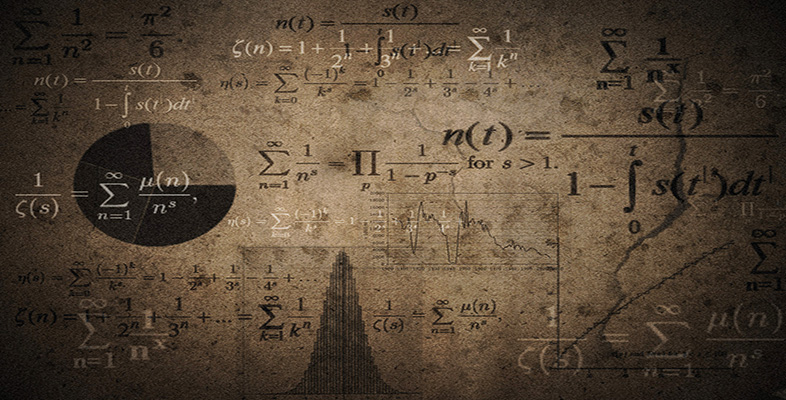Language, notation and formulas

This free course is available to start right now. Review the full course description and key learning outcomes and create an account and enrol if you want a free statement of participation.

Free course

# 3.3.1 Try some yourself

## Activity 14

If tomatoes cost 75 pence per kg, how much would 1.45 kg cost in pounds (£)?

The formula is

cost of tomatoes = (price per kilogram) × (number of kilograms).

The price per kilogram is 75 pence, and the number of kilograms is 1.45. So the formula gives

cost of tomatoes = (75 pence) × 1.45 = 108.75

Rounded to the nearest penny this is 109 pence, or £1.09.

## Activity 15

If you travel in a car at a constant speed of 60 km per hour, how far would you have travelled after 1.5 hours, after 2 hours 40 minutes and after three and a half hours?

The formula is

distance travelled = (speed) × (time).

So, after 1.5 hours,

distance travelled = (60 km per hour) × (1.5 hours) = 90 km.

As 2 hours 40 minutes is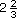hours, or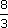hours, the formula gives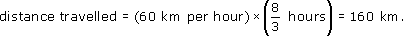For three and a half hours (3.5 hours), the formula gives

distance travelled = (60 km per hour) × (3.5 hours) = 210 km.

## Activity 16

3 Suppose you are selling a new lawn-edging strip and need to calculate the perimeters of lawns in order to quote prices to prospective customers.

The formula for the distance round the perimeter of a rectangular lawn is

perimeter = 2 × length + 2 × breadth.

Use this formula to find the perimeter of a lawn that is 10 m by 8 m.

The length of the lawn is 10 m and its breadth is 8 m. So, from the formula,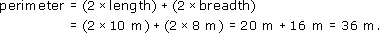The perimeter of the lawn is 36 m.

## Activity 17

Use the formulas for areas given below to find the areas of the shapes in square metres (m2).

Area square = (length)2; Area rectangle = length × breadth; Area triangle =base × height

• (a)

•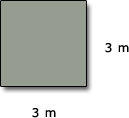•

• (b)

•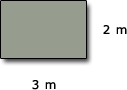•

• (c)

•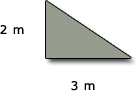• (a) Area square = (length)2 = (3 m)2 = 9 m2

• (b) Area rectangle = length × breadth = 3 m × 2 m = 6 m2

• (c) Area triangle =base × height =× 3 m × 2 m =× 6 m2 = 3 m2

MU120_4M6